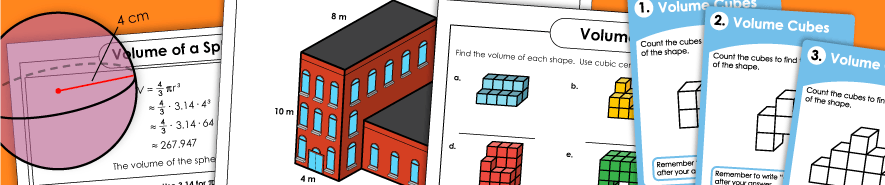# Volume Worksheets

This page has printable worksheets to help students learn about volume. With the printables listed below, students will use models and formulas to calculate the volumes of rectangular prisms, cylinders, triangular prisms, cones, and spheres.Counting Volume Cubes

These basic volume worksheets will teach students about the concept of volume as "square units." On these worksheets and task cards, students count or estimate the number of square units (blocks) shown.

Volume of Rectangular Prisms

On this page you'll find worksheets on calculating the volume of rectangular prisms. Includes basic, intermediate, and advanced level worksheets.

Volume of Composite Figures / Additive Volume (2 Rectangular Prisms)

The figures in these worksheets have composite shapes made up of 2 or more rectangular prisms combined. This skill is sometimes called additive volume.

Volume of Cylinders

Volume of Cones

These printable worksheets are designed to help you teach students to figure out the volumes of cones.

Volume of Spheres

Use these printable activities to teach students to use a formula to determine the volumes of spheres.

Area

Teach students to calculate area of rectangles, circles, trapezoids, parallelograms, as well as irregular rectilinear polygons.

Perimeter

Calculate the perimeter of polygons by adding the lengths of the sides.

Geometry

Learn about lines, segments, rays, points, coordinate planes, polygons, circles, and countless other geometry concepts.